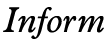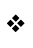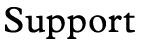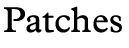Grammar property breaks in large games Submitted by: Daniel Schepler     Appeared in: Libraries long ago     Fixed in: Library 6/11 Problem If the dictionary goes above \$8000, then due to the way the Inform library determines whether the return value from a grammar property is a word or a negated word, things stop working. My fix is to change the way it determines this, to try to make it work more generally. (And in debug mode, it will warn you if this is impossible to determine reliably due to the dictionary overlapping \$8000 and an additional unfortunate coincidence.) Solution ```--- parserm.h~ Thu Dec 9 19:40:58 1999 +++ parserm.h Mon Mar 27 16:27:16 2000 @@ -391,6 +391,11 @@ Constant DEFART_PK = \$101; Constant INDEFART_PK = \$102; Global short_name_case; + +Global dict_start; +Global dict_entry_size; +Global dict_end; + ! ---------------------------------------------------------------------------- Include "language__"; ! The natural language definition, ! whose filename is taken from the ICL @@ -860,7 +865,13 @@ if (parser_trace>=2 && actor.grammar~=0 or NULL) print " [Grammar property returned ", i, "]^"; #endif; - if (i<0) { usual_grammar_after = verb_wordnum; i=-i; } + if ((i ~= 0 or 1) && + (UnsignedCompare(i, dict_start) < 0 || + UnsignedCompare(i, dict_end) >= 0 || + (i - dict_start) % dict_entry_size ~= 0)) + { usual_grammar_after = verb_wordnum; + i=-i; + } if (i==1) { results-->0 = action; results-->1 = noun; @@ -1351,7 +1362,7 @@ ! it is taken as conversation which the parser has no business in disallowing. if (actor~=player) - { if (usual_grammar_after>0) + { if (usual_grammar_after~=0) { verb_wordnum = usual_grammar_after; jump AlmostReParse; } @@ -3635,6 +3646,16 @@ standard_interpreter = \$32-->0; transcript_mode = ((0-->8) & 1); ChangeDefault(cant_go, CANTGO__TX); + dict_start = 0-->4; + dict_entry_size = dict_start->(dict_start->0 + 1); + dict_start = dict_start + dict_start->0 + 4; + dict_end = (dict_start - 2)-->0 * dict_entry_size; +#IFDEF DEBUG; + if (dict_start > 0 && dict_end < 0 && + ((-dict_start) - dict_end) % dict_entry_size == 0) + print "** Warning: grammar properties might not work + correctly **^"; +#ENDIF; buffer->0 = 120; buffer2->0 = 120;```